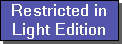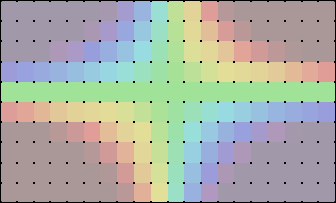The SDL Component Suite is an industry leading collection of components supporting scientific and engineering computing. Please visit the SDL Web site for more information....## NumPivotsYUnit: SDL_rchart Class: TContourPlot Declaration: property NumPivotsY: integer;

The NumPivotsY specifies the number of pivot points along the vertical axis. The number of pivot points may be set to values between 2 and 1000.

The following figure shows the pivot points on top of a colored contour surface. The property NumPivotsY has been set to 11. Thus the y-axis is divided into 10 sections (11 pivot points).Hint 1: The resolution of the contour plot may be controlled either by the properties NumPivotsX and NumPivotsY or by the property PivotResolution. The difference between these two kinds of resolution settings is that NumPivotsX and NumPivotsY allow to control the number of pivot points exactly while the property PivotResolution automatically adjusts the number of pivot points along the x- and the y-axis such that the contour plot is divided into roughly square regions.

 Hint 2: Please note that the time to calculate a contour plot is roughly proportional to the product of the properties NumPivotsX and NumPivotsY.

 Example: This method is used in the following example program (see http://www.lohninger.com/examples.html for downloading the code): contplot

Last Update: 2015-May-31